Science, Maths & Technology

### Become an OU student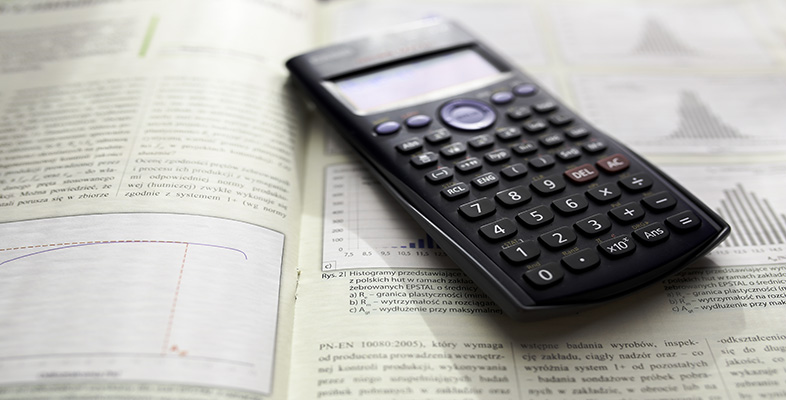Using a scientific calculator

Start this free course now. Just create an account and sign in. Enrol and complete the course for a free statement of participation or digital badge if available.

# 10 Logarithms on your calculator

Logarithms to base 10 of numbers can be found using the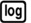key. For example,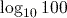can be calculated using the key sequence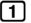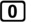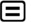. Note that as with the trigonometric functions, thekey automatically opens a bracket that must be closed if you are using the calculated logarithm as part of a longer calculation.

The second function of thekey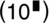, accessed using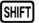, can be used as an alternative to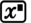when calculating powers of 10.

## Activity 19 Calculating logarithms

Use your calculator to find the values of the following.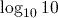,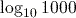,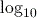(1 billion) ,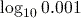All the calculations are logarithms to base 10, so we use thekey.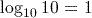,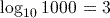,(1 billion)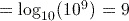,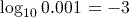.